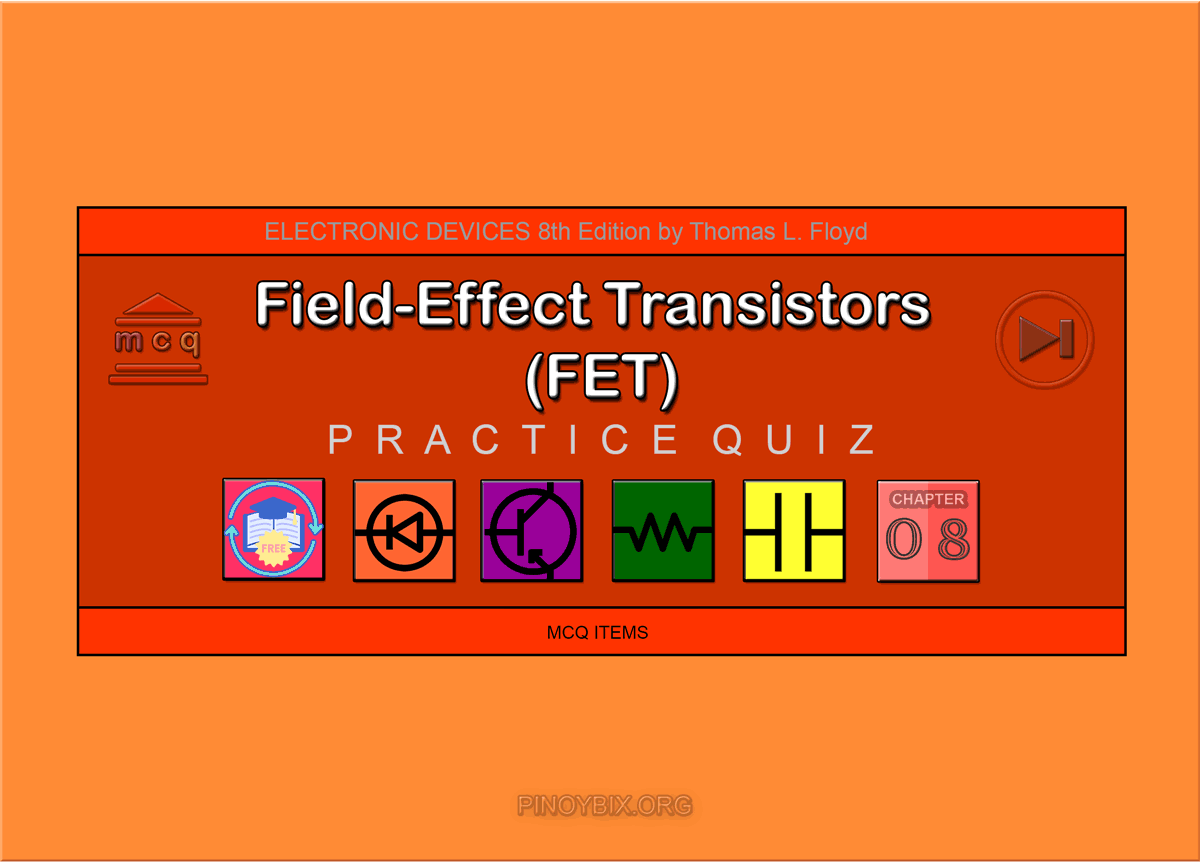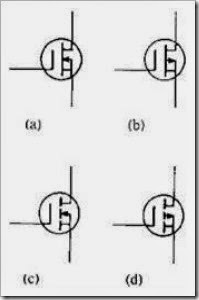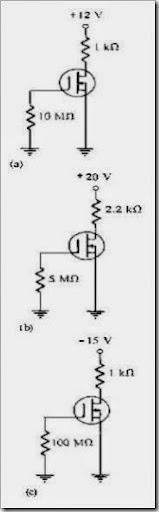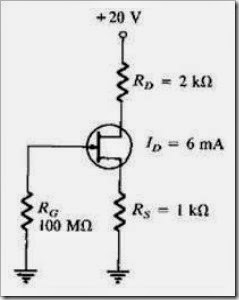# Floyd: MCQ in Field-Effect Transistors (FET)

(Last Updated On: November 30, 2019)This is the Multiple Choice Questions in Field-Effect Transistors (FET) from the book Electronic Devices – Electron Flow Version and Conventional Current Version 8th Edition by Thomas L. Floyd. If you are looking for a reviewer in Electronics Engineering this will definitely help. I can assure you that this will be a great help in reviewing the book in preparation for your Board Exam. Make sure to familiarize each and every questions to increase the chance of passing the ECE Board Exam.

#### Online Questions and Answers Topic Outline

• MCQ in Field-Effect Transistors (FET) | MCQ in JFET | MCQ in JFET Characteristics and Parameters | MCQ in JFET Biasing | MCQ in The Ohmic Region | MCQ in MOSFET | MCQ in MOSFET Characteristics and Parameters | MCQ in MOSFET Biasing | MCQ in IGBT

#### Practice Exam Test Questions

Choose the letter of the best answer in each questions.

1. On the drain characteristic curve of a JFET for VGS = 0, the pinch-off voltage is

A) below the ohmic area.

B) between the ohmic area and the constant current area.

C) between the constant current area and the breakdown region.

D) above the breakdown region.

Solution:

2. For a JFET, the value of VDS at which ID becomes essentially constant is the

A) pinch-off voltage.

B) cutoff voltage.

C) breakdown voltage.

D) ohmic voltage.

Solution:

3. The value of VGS that makes ID approximately zero is the

A) pinch-off voltage.

B) cutoff voltage.

C) breakdown voltage.

D) ohmic voltage.

Solution:

4. For a JFET, the change in drain current for a given change in gate-to-source voltage, with the drain-to-source voltage constant, is

A) breakdown.

B) reverse transconductance.

C) forward transconductance.

D) self-biasing.

Solution:

5. High input resistance for a JFET is due to

A) a metal oxide layer.

B) a large input resistor to the device.

C) an intrinsic layer.

D) the gate-source junction being reverse-biased.

Solution:

6. A dual-gated MOSFET is

A) a depletion MOSFET.

B) an enhancement MOSFET.

C) a VMOSFET.

D) either a depletion or an enhancement MOSFET.

Solution:

7. Which of the following devices has the highest input resistance?

A) diode

B) JFET

C) MOSFET

D) bipolar junction transistor

Solution:

8. A self-biased n-channel JFET has a VD = 6 V. VGS = –3 V. Find the value of VDS.

A) –3 V

B) –6 V

C) 3 V

D) 6 V

Solution:

9. Refer to Figure 8-1. Identify the p-channel E-MOSFET.Figure 8-1

A) a

B) b

C) c

D) d

Solution:

10. Refer to Figure 8-1. Identify the n-channel D-MOSFET.

A) a

B) b

C) c

D) d

Solution:

11. Refer to Figure 8-1. Identify the n-channel E-MOSFET.

A) a

B) b

C) c

D) d

Solution:

12. Refer to Figure 8-1. Identify the p-channel D-MOSFET.

A) a

B) b

C) c

D) d

Solution:

13. Refer to Figure 8-2(a). ID = 6 mA. Calculate the value of VDS.Figure 8-2

A) –6 V

B) 6 V

C) 12 V

D) –3 V

Solution:

14. Refer to Figure 8-2(b). ID = 6 mA. Calculate the value of VDS.

A) 13.2 V

B) 10 V

C) 6.8 V

D) 0 V

Solution:

15. Refer to Figure 8-2(c). ID = 6 mA. Calculate the value of VDS.

A) –9 V

B) 9 V

C) 6 V

D) –3 V

Solution:

16. A JFET data sheet specifies VGS(off) = –6 V and IDSS = 8 mA. Find the value of ID when VGS = –3 V.

A) 2 mA

B) 4 mA

C) 8 mA

D) none of the above

Solution:

17. A JFET data sheet specifies VGS(off) = –10 V and IDSS = 8 mA. Find the value of ID when VGS = –3 V.

A) 2 mA

B) 1.4 mA

C) 4.8 mA

D) 3.92 mA

Solution:

18. Refer to Figure 8-3. Determine the value of VS.Figure 8-3

A) 20 V

B) 8 V

C) 6 V

D) 2 V

Solution:

19. Refer to Figure 8-3. Calculate the value of VD.

A) 20 V

B) 8 V

C) 6 V

D) 2 V

Solution:

20. Refer to Figure 8-3. What is the value of IG?

A) 6 mA

B) 4 mA

C) 2 mA

D) 0 mA

Solution:

21. Refer to Figure 8-3. Determine the value of VGS.

A) –20 V

B) –8 V

C) –6 V

D) –2 V

Solution:

22. Refer to Figure 8-3. Calculate the value of VDS.

A) 0 V

B) 2 V

C) 4 V

D) –2 V

Solution:

23. The JFET is always operated with the gate-source pn junction ______ -biased.

A) forward

B) reverse

C) either forward or reverse

D) neither forward or reverse

Solution:

24. What three areas are the drain characteristics of a JFET (VGS = 0) divided into?

A) ohmic, constant-current, breakdown

B) pinch-off, constant-current, avalanche

C) ohmic, constant-voltage, breakdown

D) none of the above

Solution:

25. What type(s) of gate-to-source voltage(s) can a depletion MOSFET (D-MOSFET) operate with?

A) zero

B) positive

C) negative

D) any of the above

Solution:

26. The ______ has a physical channel between the drain and source.

A) D-MOSFET

B) E-MOSFET

C) V-MOSFET

D) none of the above

Solution:

27. All MOSFETs are subject to damage from electrostatic discharge (ESD).

A) true

B) false

Solution:

28. Midpoint bias for a D-MOSFET is ID = ______, obtained by setting VGS = 0.

A) IDSS/2

B) IDSS/3.4

C) IDSS

D) none of the above

Solution:

29. In a self-biased JFET circuit, if VD = VDD then ID = ______.

A) 0

B) 1

C) 2

D) cannot be determined from information above

Solution:

30. If VD is less than expected (normal) for a self-biased JFET circuit, then it could be caused by a(n)

A) open RG.

C) FET internally open at gate.

D) all of the above

Solution:

31. The resistance of a JFET biased in the ohmic region is controlled by

A) VD.

B) VGS.

C) VS.

D) VDS.

Solution:

### Complete List of Chapter MCQ in Floyd’s Electronic Devices

P inoyBIX educates thousands of reviewers and students a day in preparation for their board examinations. Also provides professionals with materials for their lectures and practice exams. Help me go forward with the same spirit.

“Will you subscribe today via YOUTUBE?”

Subscribe

PinoyBIX Engineering. © 2014-2020 All Rights Reserved | How to Donate? |